# Geometry

Geometry is the study of points, lines, planes, and anything that can be made from those three things. Geometry includes everything from angles to trapezoids to cylinders.

There is a lot of overlap with geometry and algebra because both topics include a study of lines in the coordinate plane. Geometry also provides the foundation for trigonometry, which is the study of triangles and their properties. Formulas from geometry such as area and volume are also essential for calculus.

This resource guide begins with terminology that appears throughout topics and subtopics. It then discusses angles and closed, two-dimensional shapes before moving onto three-dimensional shapes and their properties.

The subject concludes with methods for constructing geometric objects with a ruler and compass and graphing them in the coordinate plane.

## Basics of Geometry

Before moving onto other topics, it is important to brush up on geometry terms and vocabulary.

• Basic Geometry Terms
• Pairs of Lines

## Angles

An angle is formed by two rays that share a common endpoint. The distance between the two rays determines the measure of the angle. These angles form the basis of many geometric figures, most notably polygons.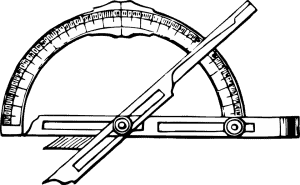This section begins with an explanation of angles and how to measure them. It then explains different types of angles and concludes with a guide for solving for an unknown angle.

## Triangles

For such simple shapes, triangles sure have a lot of properties! In fact, this topic only covers the basics of triangles. Trigonometry digs even deeper into the relationships between the sides and angles of different triangles.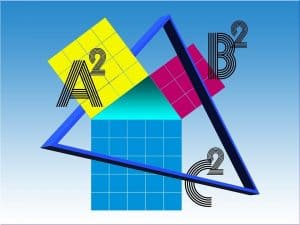This section begins with the classification of triangles and how to find their area. It then discusses the Pythagorean Theorem and its properties.

This topic also explains triangle congruency and similarity and how to determine if two triangles are congruent or similar.

The section ends with a brief introduction to sines and cosines, which will be discussed more thoroughly in trigonometry.

## Polygons

Polygons are any closed figure with straight sides. Most people tend to think of triangles, squares, pentagons, and the like when they think of polygons. Concave shapes such as plus signs and arrows, however, are also considered polygons.

A study of these figures will often focus on quadrilaterals, four-sided figures.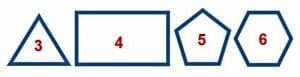This section explains polygons and their properties first. It then discusses the area of polygons generally and focuses on the area of different types of quadrilaterals.

Finally, the section explains how to find the perimeter or outside length of a polygon.

## Circles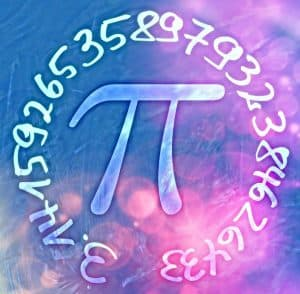Circles are not polygons because they do not have straight edges. For this reason, along with the fact that they have so many unique properties, circles get their own section. Be ready to use the ratio of a circle’s circumference to its diameter, pi!

This section includes an introduction to circles followed by an explanation of circumference. It then explains how to find the area of a circle or part of a circle. This section also explains tangents.

The topic ends by explaining how degrees and radians can both be used to measure the arc length of a circle.

## Circle Theorems

In addition to basic properties of circles, there are many unique theorems that can be applied to circles. Many of them involve tangents, secants, and objects in circles.

This section begins by defining some of the objects that will be used in circle theorems, notably chords, angles, and intercepted arcs. It then introduces several circle theorems before explaining how to apply them to different circumstances.

## Solid Geometry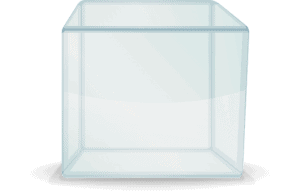After finishing with two-dimensional figures, it’s time to move on to three-dimensional figures.

Solid geometry studies all three-dimensional objects, including cubes, pyramids, and cylinders. It also focuses on the properties of these objects.

This topic begins with the volume of different types of solids and then moves on to the surface area of different solids. It also explains nets, or two-dimensional shapes that can be folded into three-dimensional ones.

## Geometric Formulas

The basic geometry formulas play an important role in upper level mathematics. Knowing how to find the area, surface area, and volume of objects is also critically important for word problems and applications.

This guide goes over those three formulas and then explains where they came from.

• Area Formula
• Surface Area Formula
• Volume Formula
• Formulas Derived
• Summary of Shapes and Formulas

## Coordinate Geometry and Graphs

Up to this point, most geometry topics have been about geometric objects in a general sense. Points, lines, and two-dimensional objects, however can be placed in the xy-plane.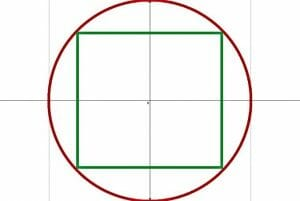This section reviews the basics of coordinate geometry from algebra, beginning with properties of coordinate planes and instructions for graphing lines. It also explains how to calculate properties of line segments, such as distance and midpoints.

The topic reviews how to work with inequalities as well as more complicated functions such as quadratic and exponential functions in the coordinate plane.

• Coordinate Geometry
• Coordinate Plane
• Slope of a Line
• Equation of a Line
• Forms of Linear Equations
• Graphing Linear Equations
• Slope and Intercept of a Linear Equation
• Explore the Straight Line Graph
• Equation of a Line Parallel to the x-axis or y-axis
• Equation of a Line Given its Slope and a Point on the Line
• Equation of a Line Given Two Points on the Lines
• Slopes of Parallel and Perpendicular Lines
• Midpoint Formula
• Distance Formula
• Graphing Linear Inequalities
• Graphing Inequalities
• Linear Programming
• Graphical Solutions of Quadratic Equations
• Graphing Cubic Functions
• Graphing Exponential Functions
• Graphing Reciprocal Functions
• Sketching the Graphs of Some Functions

## Geometric ConstructionsA compass can be used to draw a circle, and a ruler can be used to draw a straight line. With these two tools, however, one can also create many other two-dimensional shapes, find exact angles, and locate the midpoint of an angle or line.

This topic goes over the basics of geometric construction, including how to use it to make parallel and perpendicular lines. It also goes over how to make various angles and polygons with just a compass and ruler.

• Geometric Construction
• Construct and Copy a Line Segment
• Construct the Perpendicular Bisector of a Line Segment
• Construct a Perpendicular Line through a Point
• Construct Parallel Lines
• Construct a 60-Degree Angle by Constructing an Equilateral Triangle
• Construct an Angle Bisector
• Construct a 30-Degree Angle
• Construct a 45-Degree Angle
• Construct a Triangle Given SSS
• Construct a Triangle Given ASA
• Construct a Triangle Given SAS
• Construct a Parallelogram, Square, and Rectangle
• Construct Various Angles and Shapes
• Locus of a Moving Point

## Geometric Transformations

Transformations are ways of moving objects in the coordinate plane. These transformations can be described both graphically and algebraically.

This section begins by describing transformations in general before explaining translations, reflections, rotations, and dilations in greater detail.

• Math Transformations
• Translations
• Reflection
• Rotation
• Dilation